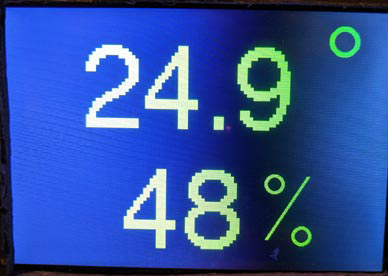# B4R TutorialUse and change font in rAdafruitGFX for TFT screen

#### derez

##### Expert
The library rAdafruitGFX is used to draw on TFT screens. It has a default font and does not give a way to change the font for drawing text.
This is what I got when drawing very large characters:To get this size I had to use size 14 in the "configure text" method...

Erel provided an inline C code to set another font:
B4X:
``````#if C
#include "FreeSans12pt7b.h"
void SetFont(B4R::Object* o) {
b4r_main::_tft->GFX->gfx->setFont(&FreeSans12pt7b);
}
#End If``````
This code is for a font named "FreeSans12pt7b.h" . If you install the library Adafruit_GFX with the Arduino IDE you get a lot of font files in a directory "Fonts" and you can copy what you want from there to rAdafruitGFX.
Change the name of the file and in the function accordingly. Replace "tft" with the name of the object you use as the adafruitGFX object and add a line to your code :
B4X:
``RunNative("SetFont", Null)``
I changed the font to FreeSans18pt7b.h and got a much better result with size 4:Next post - how to create your font.

Last edited:

#### derez

##### Expert
Sometimes the existing fonts are not what you need, for example - I wanted to show the names of the days in Hebrew on a screen doing clock.Either open one of the files in the applications directory or start a new one. On the left select the character number and letter to create, in the picture I design "א" to appear when I draw "a" .
use - defines if this letter will be included in the font file.
On the right - set the size of the character's bitmap.
Advance X sets the x place to draw the next character so it has to a little larger than the width.
Offsets define which point is the anchor so set 0 to have the top left pixel.
The characters can be of different size, for example - the letter "ו" width is much less the the others.

When all the characters you need are defined export the file as ESP8266 Adafruit to get a C font file, define your own name.
Copy the file and continue as in the first post.

With the design as above - to draw the letter "א" I'll have to code tft.GFX.DrawText("a") ...

Next post how to use the original letters (use tft.GFX.DrawText("א") to draw "א").

#### derez

##### Expert
Two more problems to solve:
1. How to use the original letters (use tft.GFX.DrawText("א") to draw "א").
2. How to work with right-to-left languishes.

To solve the first problem:
Write a function that reads each character from the string-to-draw, replace it by the character that has the new font and then recombine the string.
For example, to write tft.GFX.DrawText("ישימח") and get tft.GFX.DrawText("isimh") which will draw the correct string. (here you can see the problem of right-to-left direction).

Since the difference in ascii numbers between the required letter and the one that holds the font is not fix, I just wrote a function like this:
B4X:
``````Sub get_H(s As String) As String

If s = "א" Then Return "a"
If s = "ב" Then Return "b"
If s = "ה" Then Return "h"
If s = "ו" Then Return "v"
If s = "ח" Then Return "h"
If s = "י" Then Return "i"
If s = "ל" Then Return "l"
If s = "מ" Then Return "m"
If s = "נ" Then Return "n"
If s = "ן" Then Return "q"
If s = "ע" Then Return "o"
If s = "ר" Then Return "r"
If s = "ש" Then Return "s"
If s = "ת" Then Return "t"
Return ""
End Sub``````
To get the characters one by one seems simple but I found that the numbers for the Hebrew letters are of two bytes while the Latin are one byte, so I had to get the pairs from the Hebrew, create the string, find the replacement and add the single byte to the array.

With this procedure I also could change the order of the characters from end to start, to solve the second problem:
B4X:
``````Sub drawh(B() As Byte)
Dim n As Int = B.Length
Dim r As Int = n/2
Dim z(r), m() As Byte
Dim st As String
For i = 0 To n - 1 Step 2
st = get_H( bc.StringFromBytes(Array As Byte(B(i),B(i+1))))
m = bc.StringToBytes(st)
z(r - i/2 - 1) = m(0)  ' if order change is not needed use z(i/2) = m(0)
Next
tft.GFX.DrawText(bc.StringFromBytes(z))
End Sub``````
If I keep the order of the letters in the design I can use this code without the mapping sub:
B4X:
``````Sub drawh(B() As Byte)
Dim n As Int = B.Length
Dim r As Int = n/2
Dim z(r) As Byte
For i = 0 To n - 1 Step 2
z(r - i/2 - 1)  = B(i)+B(i+1) - 262 ' if order change is not needed use z(i/2)
Next
tft.GFX.DrawText(bc.StringFromBytes(z))
End Sub``````
262 is the difference in my case.

Now I can code drawh("חמישי") and get it drawn on the screen the same way.

B4X:
``````    tft.GFX.ConfigureText(2,tft.COLOR_RED,False)
tft.GFX.SetCursor(70,50)
drawh("ראשון")
tft.GFX.SetCursor(70,120)
drawh("רביעי")
tft.GFX.SetCursor(70,190)
drawh("חמישי")
tft.GFX.SetCursor(95,260)
drawh("שבת")
tft.GFX.SetCursor(70,330)
drawh("שלישי")``````Last edited:
•Erel and Johan Schoeman

#### derez

##### Expert
To use two or more fonts in the same application use the attached C code, call SetFontD to go back to the default:

B4X:
``````#if C
#include "FreeSans12pt7b.h"

void SetFont(B4R::Object* o) {
b4r_main::_tft->GFX->gfx->setFont(&FreeSans12pt7b);
}

void SetFontD(B4R::Object* o) {
b4r_main::_tft->GFX->gfx->setFont();
}

#End If``````

•Johan Schoeman